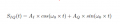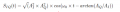# summing two signal that are 90° phase shifted(In-phase and Quadrature Signal) in xy plan?

#### TarikElec

Joined Oct 17, 2019
97
Hello everyone,
I have two signal that have two different amplitudes and have a phase shift of 90 degrees as below:then I in the book, the result is :I can imagine using vectors to achieve the first part of the fomula but I cannot find how he deduced the arc value?

Last edited:

#### Papabravo

Joined Feb 24, 2006
19,854
Use one of the cofunction identities

$$sin \;\theta\;=\;cos\left(\;\cfrac{\pi}{2}\;-\;{\theta}\;\right)$$

to convert sin to cosine, then factor the magnitudes.

•TarikElec

#### WBahn

Joined Mar 31, 2012
28,182
The claim is basically that

A·cos(φ) + B·sin(φ) = C·cos(φ-Θ)

What trig identity can you use to change the right-hand side so that it can be matched up with the terms of the left?

•TarikElec

#### TarikElec

Joined Oct 17, 2019
97
Use one of the cofunction identities

$$sin \;\theta\;=\;cos\left(\;\cfrac{\pi}{2}\;-\;{\theta}\;\right)$$

to convert sin to cosine, then factor the magnitudes.
if I consider it as x y plan and its vector. i can make the equivalent amplitude.but, the phase even with that trigonemtric conversion, I do not see the result

#### Jerry-Hat-Trick

Joined Aug 31, 2022
232

#### TarikElec

Joined Oct 17, 2019
97
Thank you guys, I will post the solution later.

#### MrAl

Joined Jun 17, 2014
9,779
Hello everyone,
I have two signal that have two different amplitudes and have a phase shift of 90 degrees as below:
View attachment 289421
then I in the book, the result is :
View attachment 289424
I can imagine using vectors to achieve the first part of the fomula but I cannot find how he deduced the arc value?

Hello there,

See if the attached drawing helps. You can identify each part by looking for the small right triangle that relates to that part.
This is for two sine waves where one has a phase shift, but you can convert that phase shift for use as a cosine wave.
Just to note, any angle in the x,y plane is always found using atan(y/x), but because that is just the main value to get the right
quadrant we have to use the two argument atan() function which is written as "atan2(y,x)" which shows the two arguments
y and x.

Here is a text version of two sine waves both with a phase shift, ph1 and ph2. You can convert this into other forms also.
This is interesting because it's an entirely different approach. There is another approach too i wont show yet.
You follow this though line by line starting with the original problem which is eq1. Notice the use of Laplace Transforms.
Each line builds on one or more previous lines.
"ev(arg1,arg2)" simply means evaluate the first argument arg1 using the values shown as the second argument arg2.
So y=x+k and then ev(y,k=1) would mean evaluate the expression for y using k=1 which would lead to y=x+1 as the result.
"numerator(arg)" simply means just return the numerator of the expression, so (y+1)/(x+1) would return just y+1. Anything more
complex has to be factored into one numerator and one denominator or just one numerator with no denominator.
"atan2(arg1,arg2)" is as described above. It's better than using atan(arg1/arg2) which does not always return the right angle.
"expand(arg)" simply means take an expression like (y+1)/(x+1) and expand it into y/(x+1)+1/(x+1).
"trigreduce(arg)" means reduce the expression according to trig identities into simplest form.

eq1:sin(t+ph1)*A+sin(t+ph2)*B; Note: this is the original problem
eq2:laplace(eq1,t,s);
eq3:factor(eq2);
eq3:numerator(eq3);

eq4:ev(eq3,s=0);
eq5:eq3-eq4;
eq5:ev(eq5,s=1);
eq6:eq4*sin(t)+eq5*cos(t);

ph:atan2(eq5,eq4);
amp:sqrt(eq4^2+eq5^2);
amp:expand(amp);
amp:trigreduce(amp);
amp:expand(amp);

res:amp*sin(t+ph);

Note there are more direct ways that are also interesting.
Have fun with it